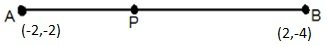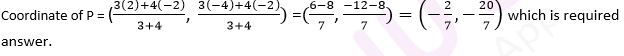Newbie

# If A and B are (-2, -2) and (2, -4), respectively, find the coordinates of P such that AP = 3/7 AB and P lies on the line segment AB. Q.8

• 0

Coordinate geometry questions of ncert  class 10th , how  i solve this question easily. Give me best solution of  coordinate geometry question of exercise 7.2 .  If A and B are (-2, -2) and (2, -4), respectively, find the coordinates of P such that AP = 3/7 AB and P lies on the line segment AB.

Share

1.